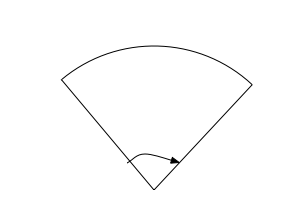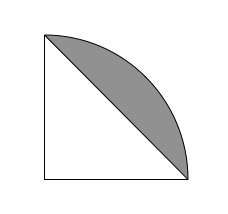Geometry

# Circles - Sector AreaIn the above sector, the central angle is $0.76$ radians and the radius is $8$. What is the area of the sector?

A circular sector with a radius of 10 has an area of 200. What is the central angle (in radians)?The above figure shows a quarter circle that is covered by a right triangle. If the radius of the circle is $16$, what is the area of the shaded region?

$\Gamma$ is a circle with radius $14$ and center $O$. $A$ and $B$ are points on $\Gamma$ such that the arc length $\widehat{AB} = 14$. What is the area of sector $AOB$?

A circular sector with central angle 5 radians has an area of 250. What is its radius?

×